﻿ RSAManaged Methods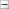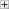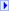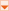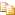The RSAManaged type exposes the following members.

#Methods

NameDescriptionClear
Releases all resources used by the AsymmetricAlgorithm class.
(Inherited from AsymmetricAlgorithm.)Decrypt(array<Byte>[]()[][])
Decrypts data with the RSA algorithm.Decrypt(array<Byte>[]()[][], RSAEncryptionPadding) (Inherited from RSA.)DecryptValue
Decrypts data with the RSA algorithm.
(Overrides RSA..::..DecryptValue(array<Byte>[]()[][]).)Dispose()()()() (Inherited from AsymmetricAlgorithm.)Dispose(Boolean)
Releases the unmanaged resources and optionally releases the managed resources.
(Overrides AsymmetricAlgorithm..::..Dispose(Boolean).)Encrypt(array<Byte>[]()[][])
Encrypts data with the RSA algorithm.Encrypt(array<Byte>[]()[][], RSAEncryptionPadding) (Inherited from RSA.)EncryptValue
Encrypts data with the RSA algorithm.
(Overrides RSA..::..EncryptValue(array<Byte>[]()[][]).)Equals
Determines whether the specified Object is equal to the current Object.
(Inherited from Object.)ExportParameters
Exports the RSAParameters.
(Overrides RSA..::..ExportParameters(Boolean).)Finalize
Allows an Object to attempt to free resources and perform other cleanup operations before the Object is reclaimed by garbage collection.
(Inherited from Object.)FromXmlString
Initializes an RSA object from the key information from an XML string.
(Inherited from RSA.)GetHashCode
Serves as a hash function for a particular type. GetHashCode()()()() is suitable for use in hashing algorithms and data structures like a hash table.
(Inherited from Object.)GetType
Gets the Type of the current instance.
(Inherited from Object.)HashData(Stream, HashAlgorithmName) (Inherited from RSA.)HashData(array<Byte>[]()[][], Int32, Int32, HashAlgorithmName) (Inherited from RSA.)ImportParameters
Imports the specified RSAParameters.
(Overrides RSA..::..ImportParameters(RSAParameters).)MemberwiseClone
Creates a shallow copy of the current Object.
(Inherited from Object.)SignData(array<Byte>[]()[][], HashAlgorithm)
Computes the hash value of the specified byte array using the specified hash algorithm, and signs the resulting hash value.SignData(array<Byte>[]()[][], HashAlgorithmName, RSASignaturePadding) (Inherited from RSA.)SignData(Stream, HashAlgorithmName, RSASignaturePadding) (Inherited from RSA.)SignData(array<Byte>[]()[][], Int32, Int32, HashAlgorithmName, RSASignaturePadding) (Inherited from RSA.)SignHash(array<Byte>[]()[][]) Obsolete.SignHash(array<Byte>[]()[][], String)
Computes the signature for the specified hash value by signing it with the private key.SignHash(array<Byte>[]()[][], HashAlgorithmName, RSASignaturePadding) (Inherited from RSA.)SignHash(RSA, array<Byte>[]()[][], String) Obsolete.ToString
Returns a String that represents the current Object.
(Inherited from Object.)ToXmlString
Creates and returns an XML string containing the key of the current RSA object.
(Inherited from RSA.)VerifyData(array<Byte>[]()[][], HashAlgorithm, array<Byte>[]()[][])
Verifies the specified signature data by comparing it to the signature computed for the specified data.VerifyData(array<Byte>[]()[][], array<Byte>[]()[][], HashAlgorithmName, RSASignaturePadding) (Inherited from RSA.)VerifyData(Stream, array<Byte>[]()[][], HashAlgorithmName, RSASignaturePadding) (Inherited from RSA.)VerifyData(array<Byte>[]()[][], Int32, Int32, array<Byte>[]()[][], HashAlgorithmName, RSASignaturePadding) (Inherited from RSA.)VerifyHash(array<Byte>[]()[][], array<Byte>[]()[][]) Obsolete.VerifyHash(array<Byte>[]()[][], String, array<Byte>[]()[][])
Verifies the specified signature data by comparing it to the signature computed for the specified hash value.VerifyHash(array<Byte>[]()[][], array<Byte>[]()[][], HashAlgorithmName, RSASignaturePadding) (Inherited from RSA.)VerifyHash(RSA, array<Byte>[]()[][], String, array<Byte>[]()[][]) Obsolete.